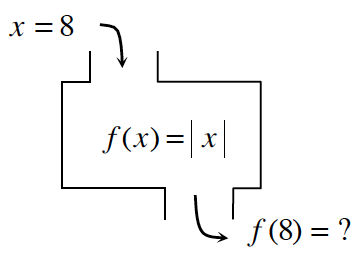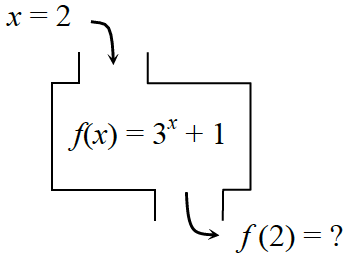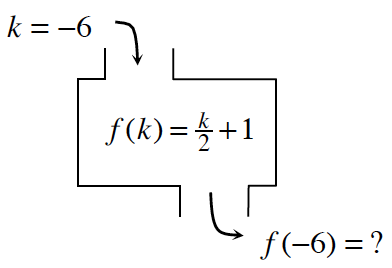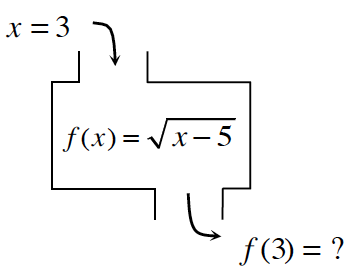### Home > GB8I > Chapter 1 Unit 1 > Lesson INT1: 1.2.1 > Problem1-38

1-38.

Determine the corresponding outputs for the given inputs of the following functions. If there is no solution, explain why not. Be careful: In some cases, there may be no solution or more than one possible solution.

1.• Substitute 8 into the equation for $x$.

$f(8) = \left|8\right| = ?$

1.• Refer to part (a).

1.• Refer to part (a).

1.• Refer to part (a).

no solution